Trigonometric Functions and Their Graphs:
The Sine and Cosine
(page 1 of 3)

Sections: The sine and cosine, The tangent, The co-functions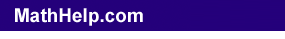At first, trig ratios related only to right triangles. Then you learned how to find ratios for any angle, using all four quadrants. Then you learned about the unit circle, in which the value of the hypotenuse was always r = 1 so that sin(θ) = y and cos(θ) = x. In other words, you progressed from geometrical figures to a situation in which there was just one input (one angle measure, instead of three sides and an angle) leading to one output (the value of the trig ratio). And this kind of relationship can be turned into a function.

Looking at the sine ratio in the four quadrants, we can take the input (the angle measure θ), "unwind" this from the unit circle, and put it on the horizontal axis of a standard graph in the x,y-plane. Then we can take the output (the value of sin(θ) = y) and use this value as the height of the function. The result looks like this: Copyright © Elizabeth Stapel 2010-2011 All Rights Reserved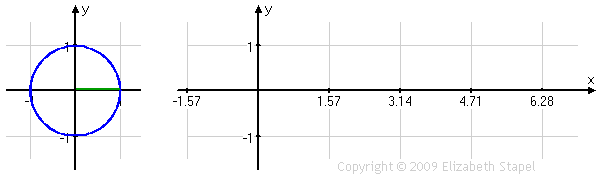As you can see, the height of the red line, being the value of sin(θ) = y, is the same in each graph. In the unit circle on the left, the angle is indicated by the green line. On the "regular" graph on the right, the angle is indicated by the scale on the horizontal axis.

If the green angle line had gone backwards, counting into negative angle measures, the horizontal graph on the right would have extended back to the left of zero. If, instead of starting over again at zero for every revolution on the unit circle, we'd counted up higher angles, then the horizontal graph on the right would have continued, up and down, over and over again, past .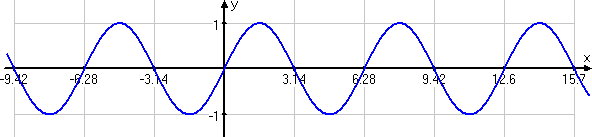The Sine Wave

From the above graph, showing the sine function from –3π to +5π, you can probably guess why this graph is called the sine "wave": the circle's angles repeat themselves with every revolution, so the sine's values repeat themselves with every length of , and the resulting curve is a wave, forever repeating the same up-and-down wave. (My horizontal axis is labelled with decimal approximations of π because that's all my grapher can handle. When you hand-draw graphs, use the exact values: π, , π/2, etc.)

When you do your sine graphs, don't try to plot loads of points. Instead, note the "important" points. The sine wave is at zero (that is, on the x-axis) at x = 0, π, and ; it is at 1 when x = π/2; it is at –1 when x = 3π/2. Plot these five points, and then fill in the curve.

Content Continues Below

We can do the same sort of function conversion with the cosine ratio: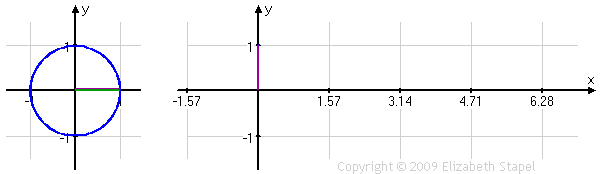The relationship is a little harder to see here, because the unit circle's line is horizontal while the standard graph's line is vertical, but you can see how those two purple lines are the same length, while the angle measure is moving from zero to . And just as with the sine graph, the cosine graph can be extended outside the interval from zero to :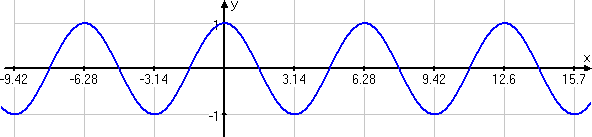The Cosine Wave

As you can see from the extended sine and cosine graphs, each curve repeats itself regularly. This trait is called "periodicity", because there is a "period" over which the curve repeats itself over and over. The length of the period for the sine and cosine curves is clearly : "once around" a circle. Also, each of sine and cosine vary back and forth between –1 and +1. The curves go one unit above and below their midlines (here, the x-axis). This value of "1" is called the "amplitude".

When you graph, don't try to plot loads of points. Note that the cosine is at y = 1 when x = 0 and ; at y = 0 for x = π/2 and 3π/2, and at y = –1 for x = π. Plot these five "interesting" points, and then fill in the curve.

Top  |  1 | 2 | 3  |  Return to Index  Next >>

 Cite this article as: Stapel, Elizabeth. "Basic Trigonometric Graphs." Purplemath. Available from     https://www.purplemath.com/modules/triggrph.htm. Accessed [Date] [Month] 2016

MathHelp.com Courses

Purplemath:

Study Skills Survey

 Copyright © 2021  Elizabeth Stapel   |   About   |   Terms of Use   |   Linking   |   Site Licensing Contact Us